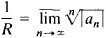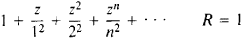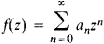# Power Series

(redirected from Power serie)
Also found in: Dictionary, Thesaurus.

## power series

[′pau̇·ər ‚sir·ēz]
(mathematics)
An infinite series composed of functions having n th term of the form an (x-x0) n , where x0 is some point and an some constant.
McGraw-Hill Dictionary of Scientific & Technical Terms, 6E, Copyright © 2003 by The McGraw-Hill Companies, Inc.
The following article is from The Great Soviet Encyclopedia (1979). It might be outdated or ideologically biased.

## Power Series

an infinite series of the form

a0 + a1z +a2z2 + . . . + anzn + . . .

where the coefficients a0, a1, a2, . . . , an, . . . are complex numbers independent of the complex variable z.

Generally speaking, the domain of convergence of a power series is an open region D = {z: ǀzǀ < R} bounded by a circle with center at z = 0. This circle is called the circle of convergence of the power series; its radius R is called the radius of convergence of the series. In the degenerate case where R = 0, the circle of convergence consists of the point z = 0. An example is 1 + l!z + 2!z2 + . . . + n!zn + . . . . If R = ∞, the domain of convergence is the entire complex plane. An example is 1 + z/1 ! + z2/2! + . . . + zn/n! + . . . . The radius of convergence of a power series is expressed in terms of its coefficients in accordance with the Cauchy-Hadamard theorem:A power series converges absolutely at all points within the circle of convergence. On the circumference of the circle, where ǀzǀ = R, the series may either converge or diverge. For example, the series 1 + z + z2 + . . . + zn + . . . , for which R = 1, diverges at each point of the circumference, where ǀzǀ = 1. On the other hand, the seriesconverges absolutely at all points of the circumference, where ǀzǀ = 1. A power series diverges at each point exterior to the circle of convergence (ǀzǀ > R).

Within the circle of convergence, the sum of the power seriesis an analytic function. Derivatives of any order of the function /(z) can be obtained by term-by-term differentiation of the series; moreover, the power series is the Taylor series of its sum.

A. A. GONCHAR

References in periodicals archive ?
In this work we have demonstrated efficiency of the Homotopy analysis transform method (Hatm) and Residual power series method (RPSM) for finding series solutions of linear and nonlinear Schrodinger equations.
The presented technique is based on Caputo and Riemann-Liouville algebras of fractional power series. Fractional differentiation and integration operators are defined using the basis of these algebras.
Abu Arqub, "Application of residual power series method for the solution of time-fractional Schroodinger equations in one-dimensional space," Fundamenta Informaticae, 2018.
In the first (Section 2), we show, for a given function, how a convergent power series is constructed out of the nonconverging one.
We can decrease the energy level using the "lowering operator" a, which differentiates any power series:
In , Hirschhorn found the 8-dissections of G(q) and its reciprocal and also proved that the sign of the coefficients in the power series expansion of G(q) and its reciprocal are periodic with period 8 and in particular that certain co-efficients are zero, a phenomenon first observed and proved by Richmond and Szekers .
For a prime p [greater than or equal to] l, we define the function [M.sub.p](q) = 1/[(q).sub.[infinity]] (h(q)- ph([q.sup.p])), then we have [M.sub.2](q) has positive power series coefficients for all positive powers of [q.sup.n] for all n except that the coefficients of [q.sup.2] and [q.sup.4] are zero.
There is a strong analogy between the classical construction of the field of real numbers and the field of power series which we are considering here.
The Power Series true on-line double-conversion technology UPS system, the latest in the range, comes with state-of-the-art PWM technology which can easily adapt to all kinds of diverse and complicated loads, such as the non-linear systems, strongly inductive or capacitive loads, discharge lamps, and induction motors.
Absolute Performance created the strength and conditioning facility using high-performance flooring, custom-built equipment from the company's elite brand AP Power Series, and renovating existing equipment.
Stay tuned to NewMoon.com to see more in the Girl Power series!

Site: Follow: Share:
Open / Close